## Göbel's Sequence

Consider the Recurrence Relation(1)

with. The first few iterates of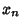are 1, 2, 3, 5, 10, 28, 154, ... (Sloane's A003504). The terms grow extremely rapidly, but are given by the asymptotic formula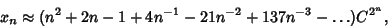(2)

where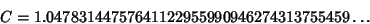(3)

(Zagier). It is more convenient to work with the transformed sequence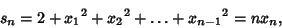(4)

which gives the new recurrence(5)

with initial condition. Now,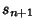will be nonintegral Iff. The smallestfor which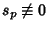(mod) therefore gives the smallest nonintegral. In addition, since,is also the smallest nonintegral.

For example, we have the sequences:(6)(7)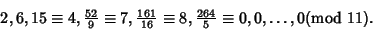(8)

Testing values ofshows that the first nonintegralis. Note that a direct verification of this fact is impossible since(9)

(calculated using the asymptotic formula) is much too large to be computed and stored explicitly.

A sequence even more striking for remaining integral over many terms is the 3-Göbel sequence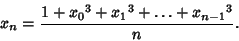(10)

The first few terms of this sequence are 1, 2, 5, 45, 22815, ... (Sloane's A005166).

The Göbel sequences can be generalized topowers by(11)

References

Guy, R. K. The Strong Law of Small Numbers.'' Amer. Math. Monthly 95, 697-712, 1988.

Guy, R. K. A Recursion of Göbel.'' §E15 in Unsolved Problems in Number Theory, 2nd ed. New York: Springer-Verlag, pp. 214-215, 1994.

Sloane, N. J. A. Sequences A003504/M0728 and A005166/M1551 in An On-Line Version of the Encyclopedia of Integer Sequences.'' http://www.research.att.com/~njas/sequences/eisonline.html and Sloane, N. J. A. and Plouffe, S. The Encyclopedia of Integer Sequences. San Diego: Academic Press, 1995.

Zaiger, D. Solution: Day 5, Problem 3.'' http://www-groups.dcs.st-and.ac.uk/~john/Zagier/Solution5.3.html.